How to Learn in 24 Hours?The Rapid Learning Movie

 Need Help? M-F: 9am-5pm(PST): Toll-Free: (877) RAPID-10 US Direct: (714) 692-2900 Int'l: 001-714-692-2900 24/7 Online Technical Support: The Rapid Support Center Secure Online Order:Need Proof? Testimonials by Our Users

 Rapid Learning Courses: MCAT in 24 Hours (2015-16) USMLE in 24 Hours (Boards) Chemistry in 24 Hours Biology in 24 Hours Physics in 24 Hours Mathematics in 24 Hours Psychology in 24 Hours SAT in 24 Hours ACT in 24 Hours AP in 24 Hours CLEP in 24 Hours DAT in 24 Hours (Dental) OAT in 24 Hours (Optometry) PCAT in 24 Hours (Pharmacy) Nursing Entrance Exams Certification in 24 Hours eBook - Survival Kits Audiobooks (MP3)

 Tell-A-Friend: Have friends taking science and math courses too? Tell them about our rapid learning system.Home »  Mathematics »  Pre-Calculus

Geometry Review

 Topic Review on "Title": Angles: An angle is either the figure formed by two rays starting from a common point or the measure of such a figure. Complimentary angles are two angles whose sum is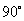. Supplementary angles are two angles whose sum is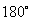. Congruent angles are angles with the same angle measurement. Vertical angles are angles at an intersection of two lines that are not adjacent to each other. Interior angles are two angles that are in the interior of a polygon. Exterior angles are two angles formed by the side of a polygon and an adjacent extends side or two angles on the outside of two parallel lines. Pythagorean Theorem: The Pythagorean Theorem is the sum of the squares for the legs of a right triangle equals the square of the hypotenuse, or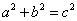. 30-60-90 Right Triangles: The sides of 30-60-90 right triangles are in the ratios of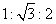. 45-45-90 Right Triangles: The sides of 45-45-90 right triangles are in the ratios of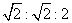. Regular polygon: A regular polygon is a geometric figure with three or more sides in which all interior angles are congruent. Area: Area is the size of a planar region or surface of a solid. Volume: Volume is the amount of space taken up by or enclosed by an object.

Rapid Study Kit for "Title":
 Flash Movie Flash Game Flash Card Core Concept Tutorial Problem Solving Drill Review Cheat Sheet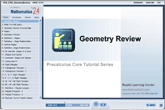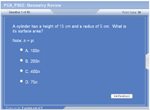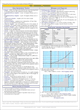"Title" Tutorial Summary : Geometry principles are covered in this tutorial. Understanding important geometric figures such as angles and their properties is important to the complete understanding of geometric relations. Calculating areas and volumes of common geometric shapes is covered in this tutorial along with their properties. The discussion of special triangle such as 45-45-90 triangles is mentioned to introduce similarity principles.

 Tutorial Features: Specific Tutorial Features: • Step by step mathematical examples showing all details: Pythagorean theorem, 30-60-90 right triangles, 45-45-90 triangles, sum of interior angles, area of complex shapes. Series Features: • Concept map showing inter-connections of new concepts in this tutorial and those previously introduced. • Definition slides introduce terms as they are needed. • Visual representation of concepts • Animated examples—worked out step by step • A concise summary is given at the conclusion of the tutorial.

 "Title" Topic List: Angles Definition of angles Angle relationships Vertical angles Interior and exterior angles Geometric Shapes and Figures Right triangles Pythagorean Theorem 30-60-90 right triangles 45-45-60 right triangles 45-45-90 right triangles Regular polygons Area and Volume Definition of area and volume Area Formulas Volume Formulas

See all 24 lessons in Pre-Calculus, including concept tutorials, problem drills and cheat sheets:
Teach Yourself Pre-Calculus Visually in 24 Hours